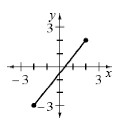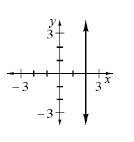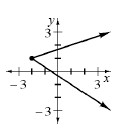Home > A2C > Chapter 1 > Lesson 1.2.3 > Problem1-110

1-110.

For each graph below, what are the domain and range?

See the Math Notes in Lesson 1.1.3.

1.Domain: $−2 ≤ x ≤ 2$
Range: $−3 ≤ y ≤ 2$

1.Domain: $x = 2$
Range: $−∞ < y < ∞$

1.